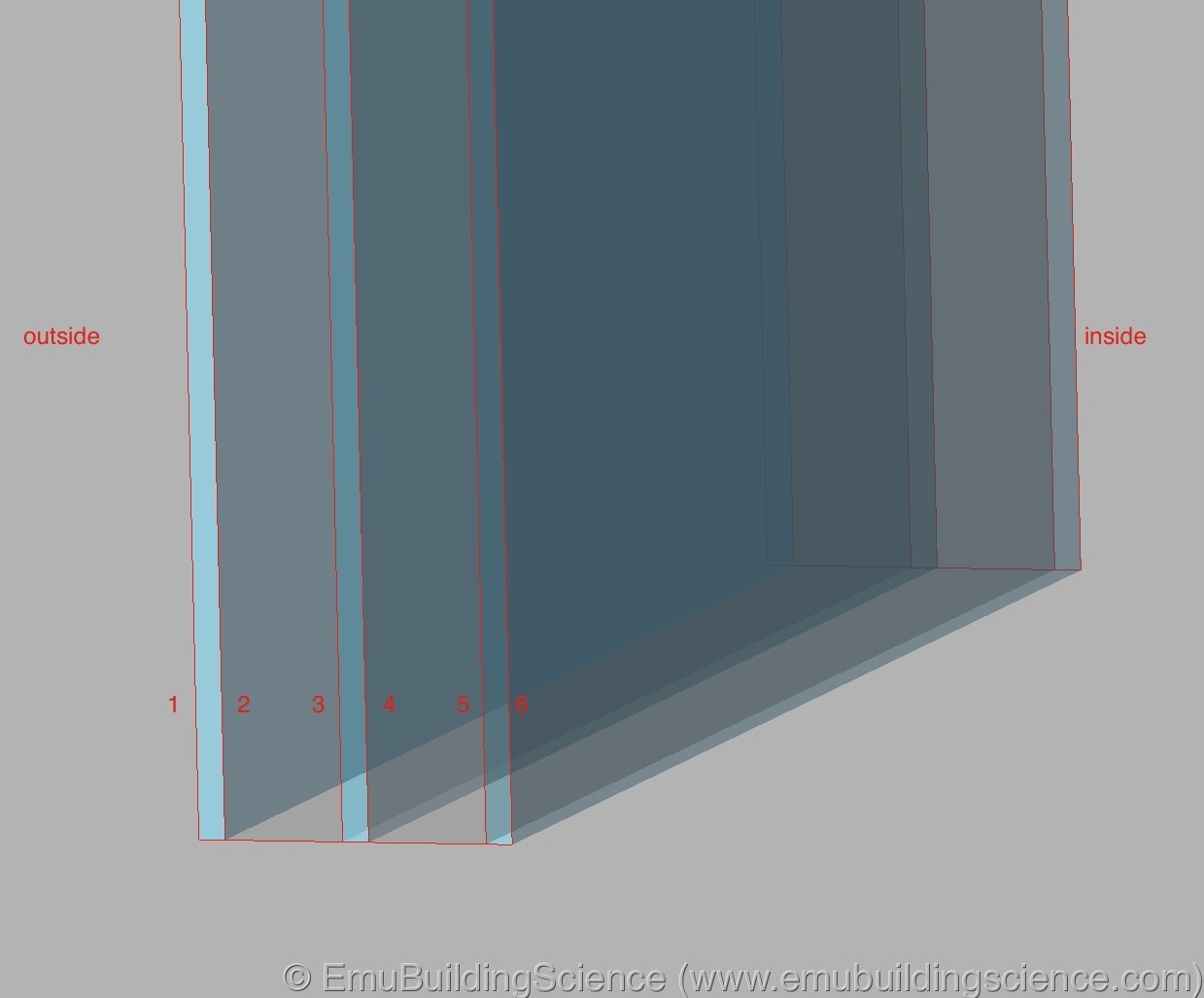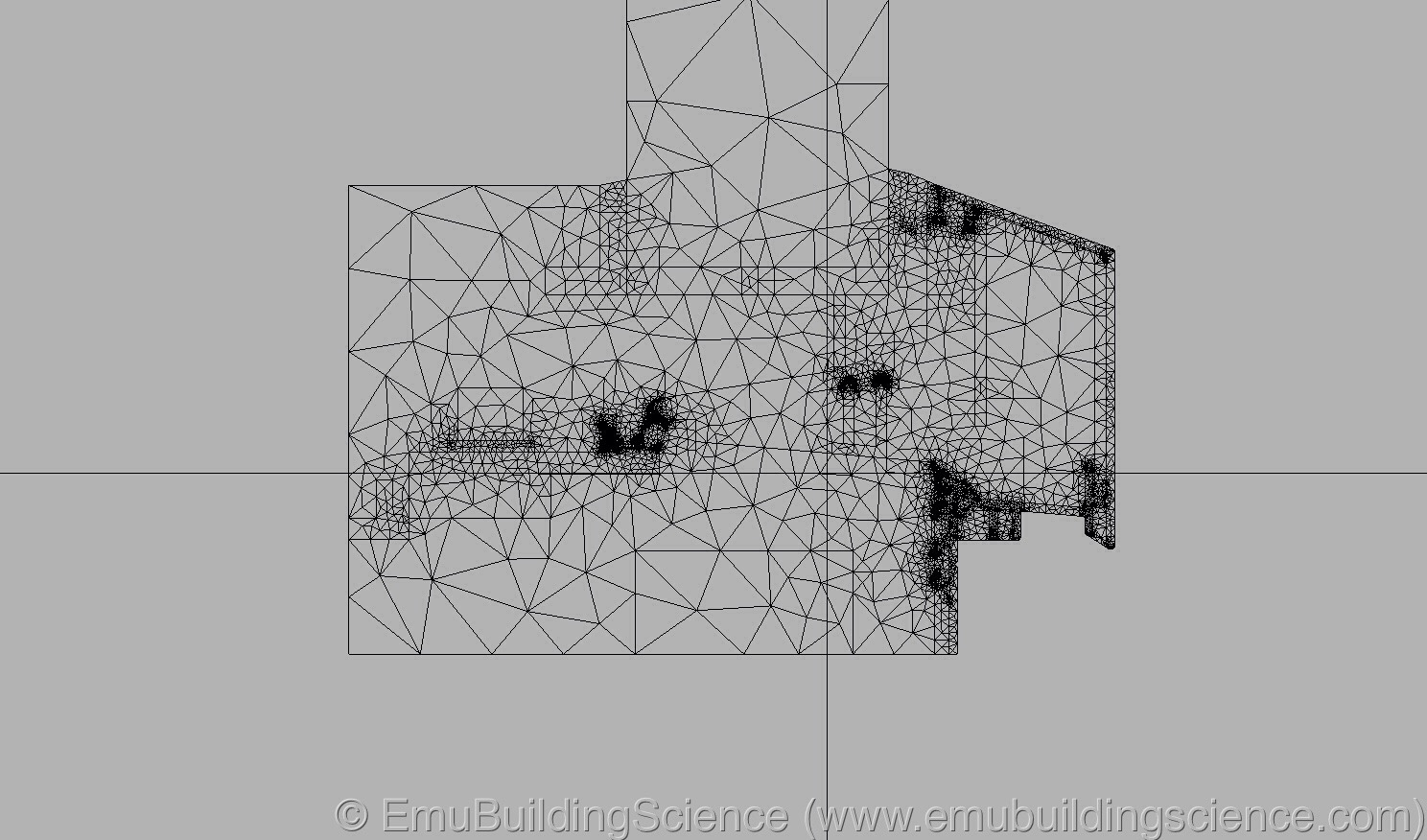# Energy efficiency of windows: the Uf value

After covering thermal transmittance U and resistance R of opaque assemblies, and insulated glass, we now cover one of the most critical areas of the thermal envelope: window frames.

Good windows are the cornerstone of a performing building for both comfort and energy efficiency, specially if it is a passive house.

In this article, we address the calculation of the thermal transmittance of the frame with computer simulation (finite elements): we’re going to cover the hot box lab test in the future, with the example of a wooden frame window.

The term “frame” used in this text refers to the entire profile, composed by the actual frame (the fixed part), and of the sash (the operable part). According to ISO10077-2, if there is a blind frame (aka buck), this is not included in the Uf calculation, and it is included in the calculation of the installation thermal bridge. We’re going to show later in this article.

#### MONODIMENSIONAL HEAT FLOW

In order to understand the Uf value of a window frame, you need to understand the U value of an opaque assembly first. Read our article here.

The U value of an opaque assembly is determined according to ISO 6946, granted that the element is composed by flat layers, parallel to each other, with little or changes in the material. For example, you cannot use this method if you have any metal profile within your assembly. The heat flow is also supposed to cross the assembly at a 90° angle.An example of an external wall: if the assembly is flat, the heat flow occurs orthogonally to the surface of the layers, in a mono dimensional way.

In the case of insulated glass units (IGUs) as well, one of the conditions required to be able to calculate the transmittance value Ug is that all panes are parallel to each other.3D model of an insulated glass unit (IGU): if the glass panes are flat and parallel to each other, the heat flow occurs in a mono dimensional way.

#### BIDIMENSIONAL HEAT FLOW

In the case of window frames, the element you intend to analyse is not composed by flat, parallel layers: the geometry is in fact very complex, including opaque elements, metal elements, and cavities.

A complex geometry determines a bidimensional heat flow, so that the thermal transmittance cannot be calculated with simple formulas.The isotherms in the bottom profile: the fact that they are curved shows that the heat flow occurs in two dimensions.This is the reason why, the Uf value of a window needs to be calculated with a finite elements software, according to ISO 10077-2.

In the US market, the National Fenstration Rating Council requires the analysis to be carried out according to ISO 15099. Please note that the results of the analysis on the same window, using ISO 10077-2 and ISO 15099 cannot be compared with each other, because they are determined with two different norm.

We’re going to explain this difference between the two norms in a specific article. In the mean time, remember that you don’t get to choose freely one or the other norm: it depends on where you need to use the number down the line (for example, PHPP requires ISO 10077-2).

Also, remember that is not a norm to make a good window: it is a good window manufacturer. Numbers are just numbers.The triangles created by the finite elements software to analyse the bottom profile of the window.

The Uf value varies according to the geometry of the frame, which dictates the bidimensional heat flow. It is very common for normal windows have to have at least two separate Uf values: one for the bottom profile, and another one for the side/top frame. If the window has two sashes, the central profile is going to have its own Uf value.

The question you may ask is:

Can you use an average Uf value?

It depends on what kind of responsibilities the designer is willing to take onto himself/herself.

A correct average Uf value is determined on the base of the correct real Uf values of the profiles, and on the geometry of the individual window. Therefore each window has its own average Uf value. The correct average Uf value does not allow for time savings.

The wrong average Uf value can be calculated on a standard 1-sash window of 1.23×1.48 m (4×5 ft). This would not take into consideration multiple sashes that may be present in the building. Even if the window manufacturer provides you with average Uf values, the responsibility of the values uses in the calculation remains with the designer of the building. As in the case of the design lambda value of a material, for the average Uf value as well the designer is free to pick any number he/she is ready to answer for.

#### THE Uf VALUE: A FICTITIOUS THERMAL TRANSMITTANCE

Let’s look at the calculation.

How do you calculate the Uf value of a window frame?

According to ISO 10077-2, you calculate the heat flow of the window frame, in the case where the glass is replaced by an hypothetical panel of insulation material (with standard lambda value of 0,035 W/mK), having the same thickness and indent of the glass unit.

The finite elements software calculates the total bidimensional heat flow, L2D, that goes through the frame and the panel.Calculationg the bidimensional heat flow for the bottom frame profile, to determine its Uf value according to ISO 10077-2.

The L2D value represents the total heat flow. From this value, you need to subtract the heat flow going through the panel (L2D_p), calculated according to its thermal transmittance (as ISO 6946), and its length bp. The length of the panel (bp) is set by the person that creates the model in the finite elements software.

L2D_p = Up * bp [W/mK or BTU/h*ft*F]

The remaining amount of heat flow (L2D_f) represents the flow that occurs through the frame:

L2D_f = L2D – L2D_p [W/mK or BTU/h*ft*F]

This value is still expressed in W/mK or BTU/h*ft*F: it shows the amount of heat (W/K or BTU/h*F) that the element exchanges with the outside per unit of its length (m or ft). To make this value “compatible” with a thermal transmittance (W/m2K or BTU/h*ft2*F), you need to refer it to the area of the frame, by dividing it by the width of the frame:

Uf = L2D_f / bf [W/m2K or BTU/h*ft2*F]

The transmittance value of the frame, Uf, is a fictitious value, that is functional to the “architecture” of the overall energy calculation. The bidimensional heat flow, L2D_f, is spread across the width of the frame, bf (as you would do with Nutella on a slice of bread).When calculating your diet (energy balance), in order to know the total calories intake (total L2D), it is not enough to know how thick is the layer of Nutella (Uf value): you also need to know how large you slice of bread is. Nutella per square meter or square foot (Nut/m2 or Nut/ft2): the unit that will make physics understandable one and for all.

This shows how the thermal transmittance of a window depends on its total heat flow, L2D_f, given by the width of the frame, bf, and its transmittance value, Uf.

Without the width value bf, the transmittance value Uf has no meaning at all.

You can use the Uf value only if you refer it to the bf value, so that the energy calculation can recreate the correct heat flow as L2D_f = Uf * bf.

To compare the energy efficiency of two different window frames, it is wrong to compare the Uf values alone. You need to compare at least their L2D_f values. A more accurate comparison is prepresented by the PSI_opaque value, used by the Passivhaus Institut to divide window frames into classes. We’re going to cover this in a specific article.

ISO 10077-2 describes what constitutes the frame of the window, so that you can determine the width of the profile (bf value).The diagram in ISO 10077-2, showing the width of the frame, bf, used to calculate the Uf value.

In case of blind frames (aka bucks, in the US), these do not belong together with the frame in the Uf calculation. Their contribution to the heat flow of the junction is calculated in the installation thermal bridge, PSI_inst.

#### CONCLUSIONS

Windows and doors have a primary role in the thermal quality of highly performing buildings and passive houses, both for comfort and energy efficiency.

In order for the overall energy design to be accurate and consistent, the designer has to calculate the transmittance values of the frames. The precision and consistency of these values with other parameters of the energy balance influence directly other values down the line, including the Uw value, installation thermal bridges and so on.

A major concern for the US market, where ISO 10077-2 and ISO 15099 coexist, is that you cannot use the results obtained by the two norms interchangeably: you need to know where you are going to use those numbers. As an example, PHPP requires ISO 10077-2; other softwares may require ISO 15099.

From the ISO 10077-2 method, it is clear that the Uf value makes sense if and only it is used with the correct value of its width, bf.

To compare the thermal performance of window frames, it is wrong to do so by the Uf value alone. You need to compare them at least by their L2D_f value, calculated as L2D_f = Uf * bf.

#### NOTE

The L2D heat flow used to determine the thermal transmittance of the window frame, Uf, was calculated with Dartwin software.#### Written by Enrico Bonilauri

Co-Founder + CPO (Chief Product Officer) @EmuBldgScience, based in Italy and Colorado. Passive house consultant, trainer, former architect.

1.passivelogical says:
1.Enrico Bonilauri says: